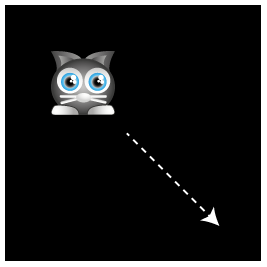## 使用速度属性

cat.vx = 0;cat.vy = 0;

vxvy设置为0表示精灵静止。

function setup() {  //Create the cat sprite  cat = new Sprite(resources["images/cat.png"].texture);  cat.y = 96;  cat.vx = 0;  cat.vy = 0;  app.stage.addChild(cat);  //Start the game loop  app.ticker.add(delta => gameLoop(delta));}function gameLoop(delta){  //Update the cat's velocity  cat.vx = 1;  cat.vy = 1;  //Apply the velocity values to the cat's  //position to make it move  cat.x += cat.vx;  cat.y += cat.vy;}